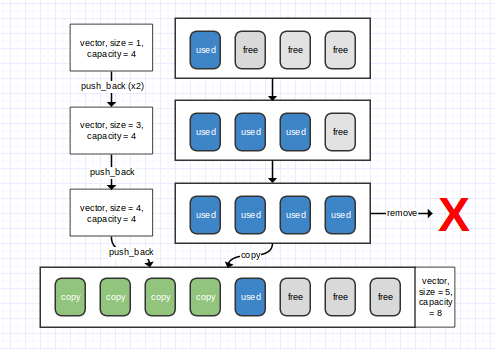# Resizing Policy of std::vector

Sometime ago when Facebook opensourced their Folly library I was reading their docs and found something interesting. In section "Memory Handling" they state

In fact it can be mathematically proven that a growth factor of 2 is rigorously the worst possible because it never allows the vector to reuse any of its previously-allocated memory
I haven't got it first time. Recently I recalled that article and decided to deal with it. So after reading and googling for a while I finally understood the idea, so I'd like to say a few words about it.

The problem is as follows: when a vector (or a similar structure with autoresize) gets filled, it should resize. It's well known that it should grow exponentially in order to preserve constant amortized complexity of insertions, but what growth factor to choose? At first glance, 2 seems to be ok — it's not so big and 2 is common for computer science :-). But it turns out that 2 is not so good. Let's take a closer look by example:Suppose we've a vector of initial size $C$. When it gets filled, we increase its size twice. We allocate memory for a vector of size $2C$ right after our original vector. So now we have vector of size $2C$ and $C$ bytes before it, where it was when it was small. Then expand it again and again and agian and so on. After $n$ expansions we'll get a vector of size $2^n C$ preceded by $C + 2C + 2^2 C + \dots 2^{n-1} C$ bytes that were occupied by this vector before.

So what's the problem? The problem is that after every increasing your vector is too big to fit previously allocated memory. How much is it bigger? Well, as we know $2^n - 1 = 1 + 2 + 4 + \dots + 2^{n-1}$, thus $2^n C - C - 2C - 2^2 C - \dots - 2^{n-1}C = C$. Therefore you have permanent lack of $C$ bytes to fit your vector in previously allocated space.

Okay, let's now solve this problem. First, let's formalize it.

Every time we increase vector of size $C$ with growing factor $k$ we do these steps:

1. Allocate $k C$ bytes
2. Create a new vector here and copy current vector's content to the new one
3. Remove the current vector, set the new one as the current

So as you can see, formula for 2 is sort of upperbound: you can not use all of previously allocated $n-1$ chunks when allocating nth, since you need to copy values from (n-1)th (though you can copy them in some temporary buffer, but it requires extra memory) chunk. So when we allocate nth chunk, we need it to be less than total free space from $n-2$ allocations: $$k^n C \le k^{n-2}C + k^{n-3}C + \dots + kC + C$$

As you can see, we can get rid of C since it's definetly positive. $$k^n \le k^{n-2} + k^{n-3} + \dots + k + 1$$

Okay, time to solve some equations! We see something like a sum of a geometric progression, and we can use a formula for it. But I don't retain it in my head, so I'll use a little trick here. Let's multiply both sides by $k-1$. We assume that $k > 1$ (it's very strange to use values greater than 1 as growth factor) $$(k-1) k^n \le (k-1) (k^{n-2} + k^{n-3} + \dots + k + 1)$$

Now we can notice that in the right side we have an expansion of $k^{n-1}-1$ (well, maybe to remember this observation is harder than remembering a formula for sum of a geometric progression…)

$$(k-1) k^n \le k^{n-1}-1$$ $$k^{n+1} - k^n \le k^{n-1}-1$$ $$k^{n+1} \le k^n + k^{n-1} - 1$$

Oh, this obstructing 1… It would be so nice if we could throw it away! Wait, but we can! If we add 1 to the right side, we will merely increase its value, so it will still suit for an upper bound (or an approximation since 1 is a constant and is very small compared to $k^n$).

$$k^{n+1} \le k^n + k^{n-1} - 1 < k^n + k^{n-1}$$ $$k^2 < k + 1$$ $$k^2 - k - 1 < 0$$

Solving this simple quadratic equation, we get $$k < \frac{1+\sqrt{5}}{2} \approx 1.61$$

So that's why growth factor in many dynamic arrays is 1.5: it is pretty big to not cause reallocations too frequently and is small enough to not use memory too extensively.

Tagged as C++, math